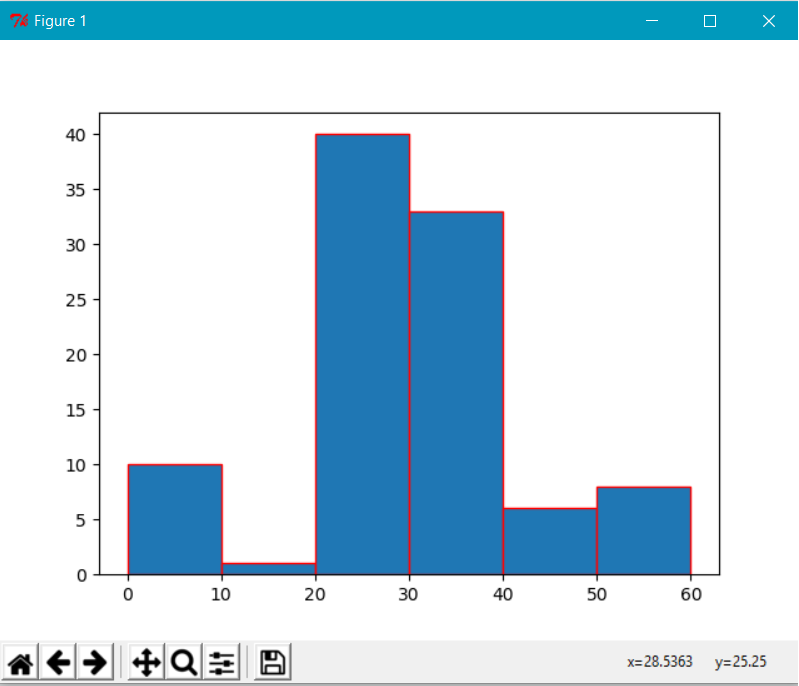# Histograms With Python

### Histograms are extremely helpful in comparing and analyzing data. This article provides the nitty-gritty of drawing a histogram using the matplotlib library in Python.

· Big Data Zone · Tutorial
Save
20.60K Views

In this article, we will look into the details of drawing a histogram using the matplotlib library. The importance of a histogram is how information is grouped together so that it can be compared and analyzed. This article provides the nitty-gritty of drawing a histogram using the matplotlib library.

A histogram is a powerful technique in data visualization. Drawing a histogram in Python is relatively easy. All you have to do is code for 3-4 lines of code. That looks pretty straightforward. But complexity is involved when we deal with live data for visualization.

In order to draw a histogram, we need to know the following concepts clearly. They are as follows:

• Axis: y-axis and x-axis.

• Data: The data can be represented as an array.

• Height and width of bars. This is determined based on the analysis. The width of the bar is called bin or intervals.

• Title of the histogram.

• Color of the bar.

• Border color of the bar.

Based on the above information, we can draw a histogram using the following code. ` `

``````import numpy as np
import matplotlib.pyplot as plt
data = [1,11,21,31,41]plt.hist([1,11,21,31,41, 51], bins=[0,10,20,30,40,50, 60], weights=[10,1,40,33,6,8], edgecolor="red")

plt.show()  ``````

This will draw a histogram, as shown below.The above dataset is uniformly distributed in this tutorial. The interval is considered as 10 in this example, which means that the x-axis is marked a width of 10 units. In the given data, the width is distributed so that each bar takes a width of 10. This means that we have grouped the data based on the bin size. Here, the size of the bin is taken as 10. However, you can change the size of this interval based on the requirements.

The histogram is drawn from the smaller values to the highest on the x- and y-axis. In this case, the smaller value of x is 1 and highest value is 51 for y.

The arguments to plot in the histogram are dataset, bin, weights, face color, and edge color.

The weight in the above arguement represents the y-axis values. Basically, the dataset, bin, and weight attributes contribute in drawing a histogram. We can calculate the value of the bin dynamically, as well. The following code snippet does this job for you:

``bins = range(min(data), max(data) + interval, interval)``

`interval` is the width that is marked on the x-axis.

In the above graph, the default color is taken as blue while drawing. In order to change the color of a histogram, we can use face color attribute in the method arguments list. The above method can be defined as below:

``````plt.hist([1,11,21,31,41, 51], bins=[0,10,20,30,40,50, 60], weights=[10,1,0,33,6,8], facecolor='y', edgecolor="red")

plt.show()``````

It will display a histogram with a yellow color.

The edge color attribute will draw a border around the bar using the color mentioned. In this case, it is `r` for red.

There are so many attributes mentioned in the matplotlib API for your perusal.

Finally, we need to give a name to the histogram by calling `plt.title("Histogram for 2018")`.

The histogram can be saved by clicking on the Save button on the GUI. Also, the following code will save the histogram as a PNG image.

``````plt.savefig("foo.png")

plt.show()
plt.close()``````

It is important to understand the order of the commands. The `savefig()` method needs to be called before the `show()` method or else it will not save the current drawing.

You can go through the matplotlib API for more data visualization support.

Topics:
big data, python, tutorial, histogram, data visualization, matplotlib

Opinions expressed by DZone contributors are their own.

Comments